Next: 6.4 Square Roots Up: 6. The Complex Numbers Previous: 6.2 Geometrical Representation   Index

# 6.3 Roots of Complex Numbers

I expect from (6.16) that every point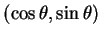in the unit circle has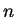th roots for all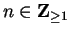, and that in fact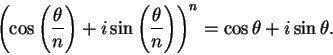In particular, each vertex of the regular-gon inscribed in the unit circle and having a vertex at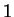will be anth root of.

6.17   Exercise. The figure below shows the seventeen points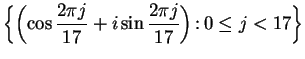.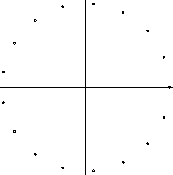Let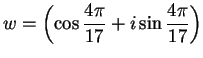and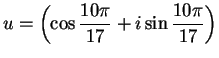. Draw the polygons-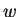-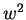-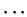-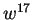and-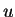-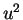--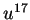on different sets of axes, (i.e. draw segments connectingto,to,,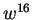to, and segnents joiningto,,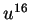to.)

6.18   Exercise. The sixth roots ofare the vertices of a regular hexagon having one vertex at. Find these numbers (by geometry or trigonometry) in terms of rational numbers or square roots of rational numbers, and verify by direct calculation that all of them do, in fact, have sixth power equal to.

6.19   Theorem (Polar decomposition.)Let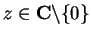. Then we can write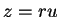whereand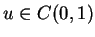. In fact this representation is unique, andI will call the representationthe polar decomposition of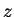, and I'll call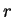the length of, and I'll callthe direction of.

Proof: Ifwhereand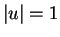, then we have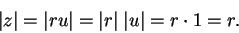This shows that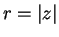, and it then follows that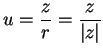. Since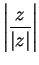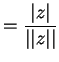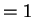, we seeand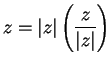gives the desired decomposition.6.20   Notation (Direction.) I will refer to any number inas a direction.

6.21   Example. The polar decomposition for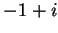is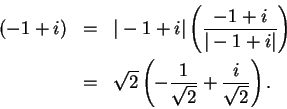I recognize from trigonometry that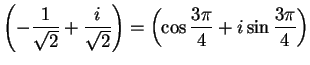.

6.22   Remark. Let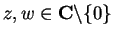. Letand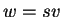be the polar decompositions of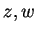, respectively, so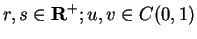. Thenwhere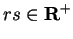and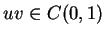. Hence we have
length of product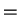product of lengths

and
direction of productproduct of directions.Next: 6.4 Square Roots Up: 6. The Complex Numbers Previous: 6.2 Geometrical Representation   Index